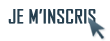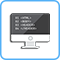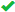IdentifiantMot de passe
Mot de passe oublié ?

Inscrivez-vous gratuitement
pour pouvoir participer, suivre les réponses en temps réel, voter pour les messages, poser vos propres questions et recevoir la newsletterDiscussion :

# Dessin de molécules disposées aléatoirement avec TikzSujet :

## Programmation (La)TeX avancée

1.Dessin de molécules disposées aléatoirement avec Tikz
Bonjour,

Je souhaite produire des molécules de diazote et de dioxygène (représentées par deux sphères accolées respectivement de couleur bleu et rouge) et les disposer aléatoirement dans un cylindre. Voici le code que j'utilise pour l'instant :
 Code : Sélectionner tout - Visualiser dans une fenêtre à part
1234567891011121314151617181920\begin{tikzpicture}
\draw [thick](-2,-2) -- (-2,2);
\draw [thick](+2,-2) -- (+2,2);
\draw [thick](-2,-2) arc (180:360:2 and 0.5);
\draw[thick,dashed] (2,-2) arc (-2:180:2 and 0.5);
\draw [thick](-2,+2) arc (180:360:2 and 0.5);
\draw [thick](+2,+2) arc (-2:180:2 and 0.5);

\pgfmathsetseed{10}
\foreach \i in {-2,-1,...,15}{
\pgfmathsetmacro{\x}{(rand*0.5 + 1)*3 - 3}
\pgfmathsetmacro{\y}{(rand*0.5 + 1)*4.7-5}
\pgfmathsetmacro{\opacVal}{rand*0.5+1}
\shade [ball color = red, opacity = \opacVal] (\x,\y) circle (0.2);}
\foreach \i in {-2,-1,...,15}{
\pgfmathsetmacro{\x}{(rand*0.5 + 1)*3 - 3}
\pgfmathsetmacro{\y}{(rand*0.5 + 1)*4.7-4.7}
\pgfmathsetmacro{\opacVal}{rand*0.5+1}
\shade [ball color = blue, opacity = \opacVal] (\x,\y) circle (0.2);}
\end{tikzpicture}
J'obtiens bien des sphères rouges et bleues disposées aléatoirement dans le cylindre, mais je n'arrive pas à obtenir les doublonds de sphères accolées.

Quelqu'un a-t-il une idée de comment modifier mon code pour obtenir le résultat souhaité ?

Merci d'avance.Répondre avec citation   0  0

2.Bonsoir,

À chaque fois que tu utilises rand, tu obtiens une nouvelle valeur aléatoire. Tu obtiens ainsi des coordonnées différentes pour tes sphères rouges et bleues.

Tu as deux solutions à disposition :
• Soit refaire appel à \pgfmathsetseed{10} entre tes deux boucles (en prenant soin de prendre la même graine). Ceci aura pour effet de remettre le générateur dans le même état que pour la première boucle, générant de ce fait exactement les mêmes valeurs.
• Soit fusionner tes deux boucles et utiliser deux variables intermédiaires \rx / \ry stockant des valeurs aléatoires fixes pour les deux sphères :
 Code : Sélectionner tout - Visualiser dans une fenêtre à part
1234567891011\pgfmathsetmacro{\rx}{(rand*0.5 + 1)*3}
\pgfmathsetmacro{\ry}{(rand*0.5 + 1)*4.7}
\pgfmathsetmacro{\opacVal}{rand*0.5+1}

\pgfmathsetmacro{\x}{\rx - 3}
\pgfmathsetmacro{\y}{\ry - 5}
\shade [ball color = red, opacity = \opacVal] (\x,\y) circle (0.2);

\pgfmathsetmacro{\x}{\rx - 3}
\pgfmathsetmacro{\y}{\ry - 4.7}
\shade [ball color = blue, opacity = \opacVal] (\x,\y) circle (0.2);Répondre avec citation   0  0

3.Bonjour,

Merci pour ton aide Winjerome. J'obtiens bien des doublons maintenant, mais je n'ai pas encore le résultat voulu. Les doublons sont tous orientés à la verticale. Je voudrais que l'orientation soit aléatoire. Est-ce possible ?Répondre avec citation   0  0

4.Oui, c'est tout à fait possible. Il te suffit de générer deux valeurs aléatoires supplémentaires : les angles formés selon les axes x et y :
 Code : Sélectionner tout - Visualiser dans une fenêtre à part
12\pgfmathsetmacro{\ax}{180 * rand}
\pgfmathsetmacro{\ay}{180 * rand}
En prenant \pgfmathsetmacro{\d}{0.3} la distance entre les deux centres des sphères, il nous reste à ajouter \d * cos(\ax) et \d * sin(\ay) aux coordonnées de la première sphère pour obtenir celles de la seconde.Répondre avec citation   0  0

5.Bonjour,

J'ai essayé ta solution Winjerome, mais je n'arrive pas à obtenir le résultat escompté. Je pense que je n'utilise pas correctement les commandes que tu m'as données. Voici ce que j'ai fait :
 Code : Sélectionner tout - Visualiser dans une fenêtre à part
12345678910111213141516\pgfmathsetseed{10}
\foreach \i in {1,2,...,10}{
\pgfmathsetmacro{\rx}{(rand*0.5 + 1)*3}
\pgfmathsetmacro{\ry}{(rand*0.5 + 1)*4.7}
\pgfmathsetmacro{\opacVal}{rand*0.5+1}
\pgfmathsetmacro{\ax}{180 * rand}
\pgfmathsetmacro{\ay}{180 * rand}
\pgfmathsetmacro{\d}{0.3}

\pgfmathsetmacro{\x}{\rx - 3}
\pgfmathsetmacro{\y}{\ry - 5}
\shade [ball color = red, opacity = \opacVal] (\x+\d * cos(\ax),\y+\d * sin(\ay)) circle (0.2);

\pgfmathsetmacro{\x}{\rx - 3}
\pgfmathsetmacro{\y}{\ry - 4.7}
\shade [ball color = red, opacity = \opacVal] (\x,\y) circle (0.2);}
Peux-tu me dire ce qui ne va pas ?Répondre avec citation   0  0

6.Attention de bien mettre la partie calcul au sein des macros \pgfmathsetmacro. Tu ne peux pas en faire au sein des coordonnées de \shade.
Au passage \d étant fixe, tu peux le sortir de la boucle.

Pour le reste, tu as juste à partir de coordonnées communes. Mettons (\rx, \ry). Pour les premières coordonnées, tu ne touches rien :
 Code : Sélectionner tout - Visualiser dans une fenêtre à part
\shade [ball color = red, opacity = \opacVal] (\rx,\ry) circle (0.2);
Pour les secondes, tu ajoutes les angles aléatoires :
 Code : Sélectionner tout - Visualiser dans une fenêtre à part
123\pgfmathsetmacro{\x}{\rx + \d * cos(\ax)}
\pgfmathsetmacro{\y}{\ry + \d * sin(\ay)}
\shade [ball color = blue, opacity = \opacVal] (\x,\y) circle (0.2);Répondre avec citation   0  0

7.Du coup, j'obtiens bien des molécules avec des orientations différentes, mais elles ne sont plus confinées dans le cylindre. Du coup, j'ai essayé d'écrire ceci :

 Code : Sélectionner tout - Visualiser dans une fenêtre à part
12345678910111213141516171819202122232425262728\begin{tikzpicture}
%Dessin cylindre
\draw [thick](-2,-2) -- (-2,2);
\draw [thick](+2,-2) -- (+2,2);
\draw [thick](-2,-2) arc (180:360:2 and 0.5);
\draw[thick,dashed] (2,-2) arc (-2:180:2 and 0.5);
\draw [thick](-2,+2) arc (180:360:2 and 0.5);
\draw [thick](+2,+2) arc (-2:180:2 and 0.5);

%Dessin des molécules
\pgfmathsetseed{10}
\pgfmathsetmacro{\d}{0.29}
\foreach \i in {1,2,...,10}{
\pgfmathsetmacro{\rx}{(rand*0.5 + 1)*3}
\pgfmathsetmacro{\ry}{(rand*0.5 + 1)*4.7}
\pgfmathsetmacro{\opacVal}{rand*0.5+1}
\pgfmathsetmacro{\ax}{180 * rand}
\pgfmathsetmacro{\ay}{180 * rand}

\pgfmathsetmacro{\x}{\rx - 3}
\pgfmathsetmacro{\y}{\ry - 5}
\shade [ball color = red, opacity = \opacVal] (\rx,\ry) circle (0.2);

\pgfmathsetmacro{\x}{\rx + \d * cos(\ax)-3} % J'ai ajouté le -3 qui était présent dans le code précédent.
\pgfmathsetmacro{\y}{\ry + \d * sin(\ay)-4.7}% Ici j'ai ajouté le -4.7
\shade [ball color = red, opacity = \opacVal] (\x,\y) circle (0.2);}
\end{tikzpicture}
Néanmoins, quand je fais les deux ajouts énoncés ci-dessus, je perds mes doublons de molécules et seules la moitié des sphères viennent se replacer dans le cylindre ; les autres restent en dehors. Pourtant sans les angles, ce code fonctionnait : du coup, je ne vois pas ce qui bloque...Répondre avec citation   0  0

8.Envoyé par RP766Code : Sélectionner tout - Visualiser dans une fenêtre à part
12% J'ai ajouté le -3 qui était présent dans le code précédent.
% Ici j'ai ajouté le -4.7
Justement non !

Le décalage, on l'introduit dorénavant avec les angles. Si toi tu ajoutes en plus ce décalage de -5 sur l'un et -4.7 sur l'autre, que penses-tu qu'il arrive ?

Je répète : pars des mêmes coordonnées. Si tu veux appliquer un décalage, fais-le à la génération des coordonnées d'origine : (\rx, \ry). Ne touche pas au reste.Répondre avec citation   0  0

9.Ok, merci pour ta patience ; j'ai réussi à obtenir le résultat souhaitéRépondre avec citation   0  0

+ Répondre à la discussion
Cette discussion est résolue.
 Actualités FAQs LaTeX Tutoriels LaTeX Livres LaTeX